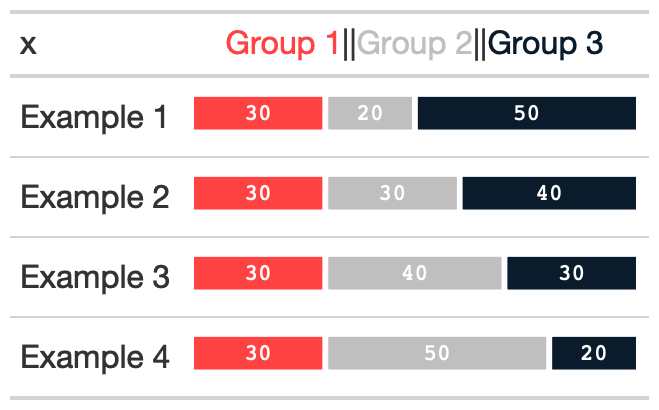The gt_plt_bar_stack function takes an existing gt_tbl object and converts the existing values into a percent stacked barchart. The bar chart will represent either 2 or 3 user-specified values per row, and requires a list column ahead of time. The palette and labels need to be equal length. The values must either add up to 100 ie as percentage points if using position = 'fill', or can be raw values with position = 'stack'. Note that the labels can be controlled via the fmt_fn argument and the scales::label_???() family of function.

## Usage

gt_plt_bar_stack(
gt_object,
column = NULL,
palette = c("#ff4343", "#bfbfbf", "#0a1c2b"),
labels = c("Group 1", "Group 2", "Group 3"),
position = "fill",
width = 70,
fmt_fn = scales::label_number(scale_cut = cut_short_scale(), trim = TRUE)
)

## Arguments

gt_object

An existing gt table object of class gt_tbl

column

The column wherein the percent stacked barchart should replace existing data. Note that the data must be represented as a list of numeric values ahead of time.

palette

A color palette of length 2 or 3, represented either by hex colors ("#ff4343") or named colors ("red").

labels

A vector of strings of length 2 or 3, representing the labels for the bar chart, will be colored according to the palette as well.

position

An string indicator passed to ggplot2 indicating if the bar should be a percent of total "fill" or stacked as the raw values "stack".

width

An integer representing the width of the bar chart in pixels.

fmt_fn

A specific function from scales::label_??? family. Defaults to scales::label_number()

## Value

An object of class gt_tbl.

## Examples

library(gt)
library(dplyr)

ex_df <- dplyr::tibble(
x = c("Example 1","Example 1",
"Example 1","Example 2","Example 2","Example 2",
"Example 3","Example 3","Example 3","Example 4","Example 4",
"Example 4"),
measure = c("Measure 1","Measure 2",
"Measure 3","Measure 1","Measure 2","Measure 3",
"Measure 1","Measure 2","Measure 3","Measure 1","Measure 2",
"Measure 3"),
data = c(30, 20, 50, 30, 30, 40, 30, 40, 30, 30, 50, 20)
)

tab_df <- ex_df %>%
group_by(x) %>%
summarise(list_data = list(data))

tab_df

ex_tab <- tab_df %>%
gt() %>%
gt_plt_bar_stack(column = list_data)Other Plotting: gt_plt_bar_pct(), gt_plt_bar(), gt_plt_dist(), gt_plt_percentile(), gt_plt_point(), gt_plt_sparkline(), gt_plt_winloss()